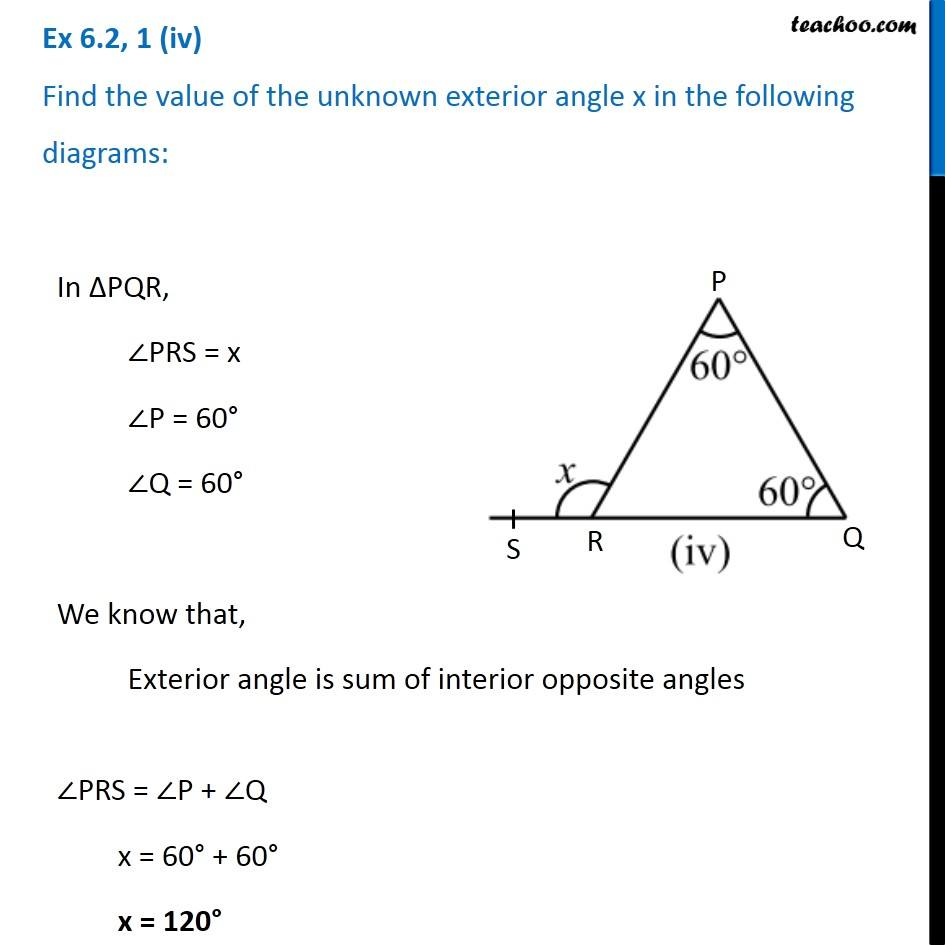1. Chapter 6 Class 7 Triangle and its Properties
2. Serial order wise
3. Ex 6.2

Transcript

Ex 6.2, 1 (iv) Find the value of the unknown exterior angle x in the following diagrams:In ∆PQR, ∠PRS = x ∠P = 60° ∠Q = 60° We know that, Exterior angle is sum of interior opposite angles ∠PRS = ∠P + ∠Q x = 60° + 60° x = 120°